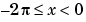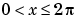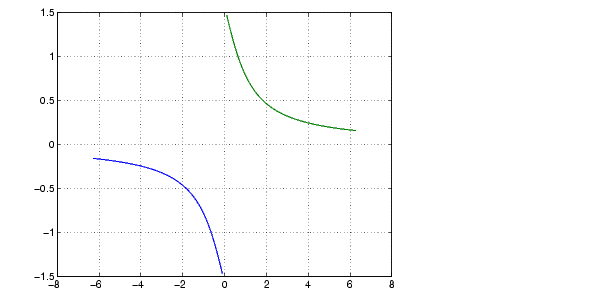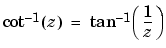MATLAB Function Referenceacot

Inverse cotangent, result in radians

Syntax

• ```Y = acot(X)
```

Description

```Y = acot(X) ``` returns the inverse cotangent (arccotangent) for each element of `X`.

The `acot` function operates element-wise on arrays. The function's domains and ranges include complex values. All angles are in radians.

Examples

Graph the inverse cotangent over the domainsand.

• ```x1 = -2*pi:pi/30:-0.1;
x2 = 0.1:pi/30:2*pi;
plot(x1,acot(x1),x2,acot(x2)), grid on```

Definition

The inverse cotangent can be defined as

•Algorithm

`acot` uses FDLIBM, which was developed at SunSoft, a Sun Microsystems, Inc. business, by Kwok C. Ng, and others. For information about FDLIBM, see http://www.netlib.org.

See Also

`cot`, `acotd`, `acoth`

© 1994-2005 The MathWorks, Inc.